Test Description

## 20 Questions MCQ Test Mathematics (Maths) Class 8 | Olympiad Test: Rational Numbers

Olympiad Test: Rational Numbers for Class 8 2023 is part of Mathematics (Maths) Class 8 preparation. The Olympiad Test: Rational Numbers questions and answers have been prepared according to the Class 8 exam syllabus.The Olympiad Test: Rational Numbers MCQs are made for Class 8 2023 Exam. Find important definitions, questions, notes, meanings, examples, exercises, MCQs and online tests for Olympiad Test: Rational Numbers below.
Solutions of Olympiad Test: Rational Numbers questions in English are available as part of our Mathematics (Maths) Class 8 for Class 8 & Olympiad Test: Rational Numbers solutions in Hindi for Mathematics (Maths) Class 8 course. Download more important topics, notes, lectures and mock test series for Class 8 Exam by signing up for free. Attempt Olympiad Test: Rational Numbers | 20 questions in 20 minutes | Mock test for Class 8 preparation | Free important questions MCQ to study Mathematics (Maths) Class 8 for Class 8 Exam | Download free PDF with solutions
 1 Crore+ students have signed up on EduRev. Have you?
Olympiad Test: Rational Numbers - Question 1

### Which of the following statements is true?

Detailed Solution for Olympiad Test: Rational Numbers - Question 1

Every rational number is not a fraction. In rational numbers, we use integers and in fractions, we use only natural numbers.

Olympiad Test: Rational Numbers - Question 2

### Compare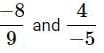Detailed Solution for Olympiad Test: Rational Numbers - Question 2

Expressing in standard form, we get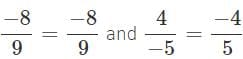Express each rational number with L.C.M as denominator. L.C.M of 9 and 5 is 9 × 5 = 45.

∴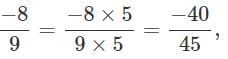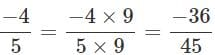Comparing the numerators, we have −40<−36 .

∴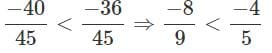Olympiad Test: Rational Numbers - Question 3

### How is -28/84 expressed as a rational number with numerator 4?

Detailed Solution for Olympiad Test: Rational Numbers - Question 3

Given, -28/84
Dividing both the numbers by 7.
Therefore, -4/12.

Olympiad Test: Rational Numbers - Question 4

17/11 - 6/11

Detailed Solution for Olympiad Test: Rational Numbers - Question 4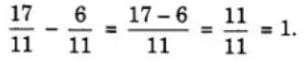Olympiad Test: Rational Numbers - Question 5

Which of the following statements is false?

Detailed Solution for Olympiad Test: Rational Numbers - Question 5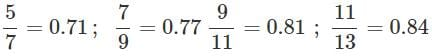So,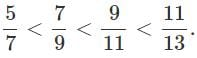Hence, both (b) and (c) are false.

Olympiad Test: Rational Numbers - Question 6

Which of the following is a rational number between 1/4 and 1/3 ?

Detailed Solution for Olympiad Test: Rational Numbers - Question 6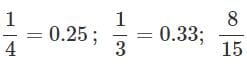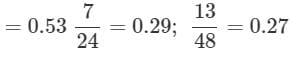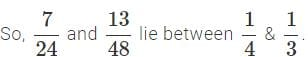Olympiad Test: Rational Numbers - Question 7

Which of the following is the correct arrangement of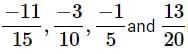in descending order?

Detailed Solution for Olympiad Test: Rational Numbers - Question 7

LCM of 5,10,15 and 20 which is 60. Now making the denominator same, we have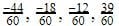. So descending order is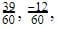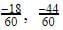that is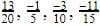Olympiad Test: Rational Numbers - Question 8

Simplify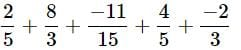Detailed Solution for Olympiad Test: Rational Numbers - Question 8

LCM of 5,3 and 15 is 15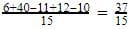Olympiad Test: Rational Numbers - Question 9

What is the sum of the additive inverse and multiplicative inverse of 2?

Detailed Solution for Olympiad Test: Rational Numbers - Question 9

Additive inverse of 2 is −2
Multiplicative inverse of 2 is 1/2
∴ The sum of multiplicative inverse and additive inverse =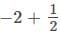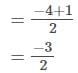Answer :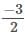Olympiad Test: Rational Numbers - Question 10

Subtract 2/3 from 7/8

Detailed Solution for Olympiad Test: Rational Numbers - Question 10

Solution :- 7/8 - 2/3

= {(21-16)/24}

= 5/24

Olympiad Test: Rational Numbers - Question 11

Which among the following is a rational number equivalent to -5/-3 ?

Detailed Solution for Olympiad Test: Rational Numbers - Question 11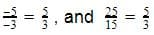Hence the correct answer is 5/3.

Olympiad Test: Rational Numbers - Question 12

The value of (5/4) – (8/3) is:

Detailed Solution for Olympiad Test: Rational Numbers - Question 12

5/4 – 8/3

Making the denominator equal:

[(5/4) x (3/3)] – [(8/3) x (4/4)]

= (15/12) – (32/12)

= (15 – 32)/12

= -17/12

Olympiad Test: Rational Numbers - Question 13

Which number is in the middle if -1/6, 4/9, 6/-7, 2/5 and -3/4 arranged in descending order?

Detailed Solution for Olympiad Test: Rational Numbers - Question 13

-⅙ = -0.16

4/9 = 0.44

-6/7 = -0.85

⅖ = 0.40

-¾ = -0.75

Descending Order : - 4/9, 2/5, -1/6, -3/4, -6/7

Middle term : - -1/6

Olympiad Test: Rational Numbers - Question 14

What is the average of the two middle rational numbers when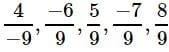and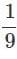are arranged in ascending order?

Detailed Solution for Olympiad Test: Rational Numbers - Question 14

Arranging the given rational numbers in ascending order, we  get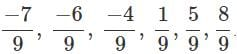The two middle rational numbers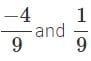Their average is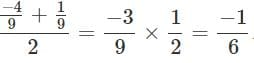Olympiad Test: Rational Numbers - Question 15

Subtract -5/9 from -3/7.

Detailed Solution for Olympiad Test: Rational Numbers - Question 15

(-3*9 + 5*7 )/63

8/63

Olympiad Test: Rational Numbers - Question 16

What number should be added to -4/7 to get 5/9 ?

Detailed Solution for Olympiad Test: Rational Numbers - Question 16

Let the number be x

Then,

X+(-4/7)=5/9

X=5/9+4/7

X= 35+36/63

X=71/63

Olympiad Test: Rational Numbers - Question 17

What should be subtracted from -7/11 to get −2?

Detailed Solution for Olympiad Test: Rational Numbers - Question 17

Let x be subtracted from -7/11
then -7/11 - x= -2
-x= -2 + (7/11)
-x= -2 + 7/11
-x= -15/11
x= 15/11

Olympiad Test: Rational Numbers - Question 18

By what rational number should -3/5 be multiplied to obtain 21?

Detailed Solution for Olympiad Test: Rational Numbers - Question 18

Let the rational no be x
-3/5 X x = 21
x = (21 X5 )/ -3
x = -35

Olympiad Test: Rational Numbers - Question 19

The sum of two rational numbers is −3. If one of them is -11/5, find the other.

Detailed Solution for Olympiad Test: Rational Numbers - Question 19

Let the other number be x

x+(-11/5)= -3

x= -3+11/5

x=-15+11/5

x= -4/5

Olympiad Test: Rational Numbers - Question 20

Which of the following is the resultant of 1/12 + -1/3 + -1/6 + -5/7 + 3/4 + 10/7?

Detailed Solution for Olympiad Test: Rational Numbers - Question 20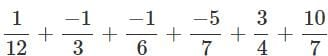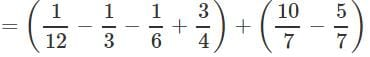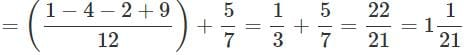## Mathematics (Maths) Class 8

193 videos|360 docs|48 tests
 Use Code STAYHOME200 and get INR 200 additional OFF Use Coupon Code